## 7.10Speciﬁc dissipation rate

The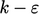model is one of a family of two-equation models for turbulence. With two equations, the models can represent each of the scales,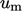and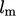, which characterise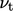. Most often,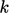is used to represent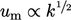.

The other variable must represent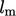and so far we have used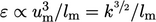with SI units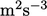. The speciﬁc dissipation rate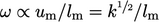, with SI units of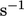, is a popular alternative for this variable in turbulence modelling.

While Kolmogorov ﬁrst proposed a two-equation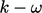model,15 the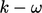models used in CFD originate from Wilcox.16 Here, “models” is plural since there are several versions of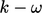model with modiﬁcations and additions from its original form.

The originalmodel is presented below (with some changes to the original variable names), assuming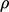= constant for direct comparison with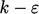in Sec. 7.1 .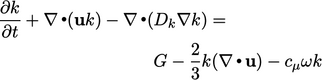(7.36)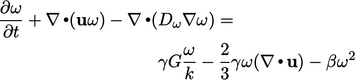(7.37)

The standard model coeﬃcients are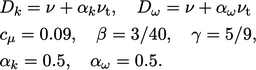(7.38)
Comparing dissipation terms in Eq. (7.1 ) and Eq. (7.36) gives the relation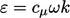. Substituting in Eq. (6.31 ) leads to a simple relation for turbulent viscosity, given by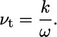(7.39)
Inlet and initial estimates for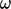can be calculated by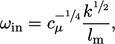(7.40)
using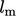, in a manner similar to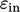in Eq. (7.4 ).

With wall functions, the boundary condition applied toset a near-wall cell value according to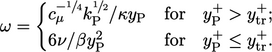(7.41)
The expression for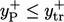(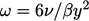) is a solution to the following equation for the viscous sub-layer where diﬀusion and dissipation terms dominate in Eq. (7.37 ):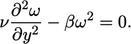(7.42)
The equivalent dissipation terms for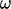in Eq. (7.37) and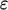in Eq. (7.2 ) are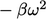and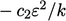respectively. The former is more stable in a numerical solution since it is insensitive to variations in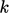.
15Andrey Nikolaevich Kolmogorov, Equations of turbulent motion in an incompressible ﬂuid, ﬁrst published in Russian in Izv. Akad. Nauk SSSR 6, 1941.
16David Wilcox, Reassessment of the scale-determining equation for advanced turbulence models, 1988.

Notes on CFD: General Principles - 7.10 Speciﬁc dissipation rate